#### Obtaining a state transition equation

Value iterations occur over discrete stages; however, the integral curves of feedback plans occur over continuous time. Therefore, the time interval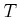needs to be sampled. Let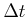denote a small positive constant that represents a fixed interval of time. Let the stage indexrefer to time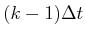. Now consider representing a velocity field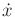over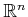. By definition,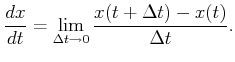(8.61)

In Section 8.3.1, a velocity field was defined by assigning some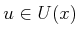to each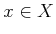. If the velocity vectoris integrated from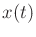over a small, then a new state,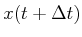, results. Ifremains constant, then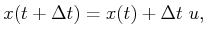(8.62)

which is called an Euler approximation. If a feedback plan is executed, thenis determined fromvia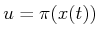. In general, this means thatcould vary as the state is integrated forward. In this case, (8.62) is only approximate,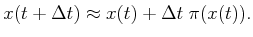(8.63)

The expression in (8.62) can be considered as a state transition equation that works over stages. Let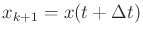and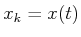. The transitions can now be expressed as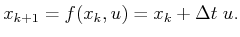(8.64)

The quality of the approximation improves asdecreases. Better approximations can be made by using more sample points along time. The most widely known approximations are the Runge-Kutta family. For optimal motion planning, it turns out that the direction vector almost always remains constant along the integral curve. For example, in Figure 8.13d, observe that piecewise-linear paths are obtained by performing gradient descent of the optimal navigation function. The direction vector is constant over most of the resulting integral curve (it changes only as obstacles are contacted). Therefore, approximation problems tend not to arise in motion planning problems. When approximating dynamical systems, such as those presented in Chapter 13, then better approximations are needed; see Section 14.3.2. One important concern is thatis chosen in a way that is compatible with the grid resolution. Ifis so small that the actions do not change the state enough to yield new interpolation neighbors, then the interpolated cost-to-go values will remain constant. This implies thatmust be chosen to ensure thathas a different set of interpolation neighbors than.

An interesting connection can be made to the approximate motion planning problem that was developed in Section 7.7. Formulation 7.4 corresponds precisely to the approximation defined here, except that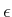was used instead ofbecause velocities were not yet considered (also, the initial condition was specified because there was no feedback). Recall the different possible action spaces shown in Figure 7.41. As stated in Section 7.7, if the Manhattan or independent-joint models are used, then the configurations remain on a grid of points. This enables discrete value iterations to be performed. A discrete feedback plan and navigation function, as considered in Section 8.2.3, can even be computed. If the Euclidean motion model is used, which is more natural, then the transitions allow a continuum of possible configurations. This case can finally be handled by using interpolation over the configuration space, as described in this section.

Steven M LaValle 2020-08-14